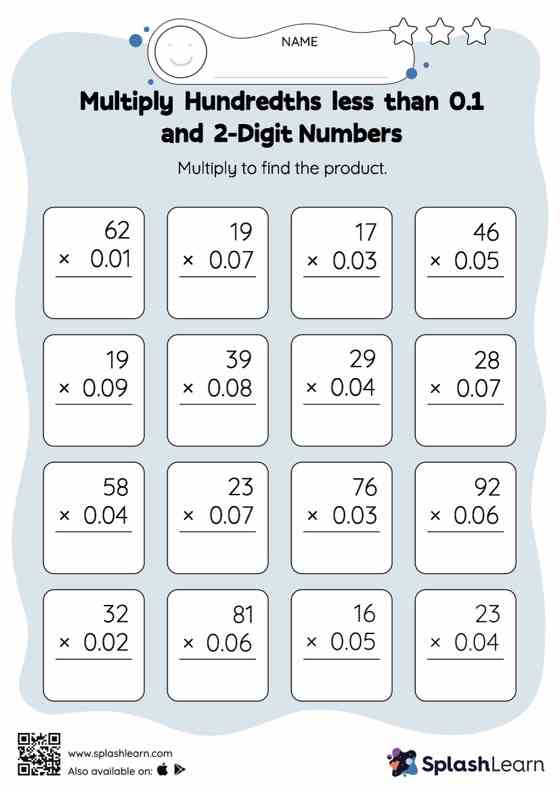# Multiply Hundredths less than 0.1 and 2-Digit Numbers: Vertical Multiplication Worksheet

Home > Multiply Hundredths less than 0.1 and 2-Digit Numbers: Vertical MultiplicationGive your little one some practice building multiplication skills with this multiply hundredths less than 0.1 And 2-digit numbers worksheet. In each problem, the numbers are laid out in the vertical format. In this format, pair of digits in each successive place are tackled one by one. This helps students follow a structured approach.